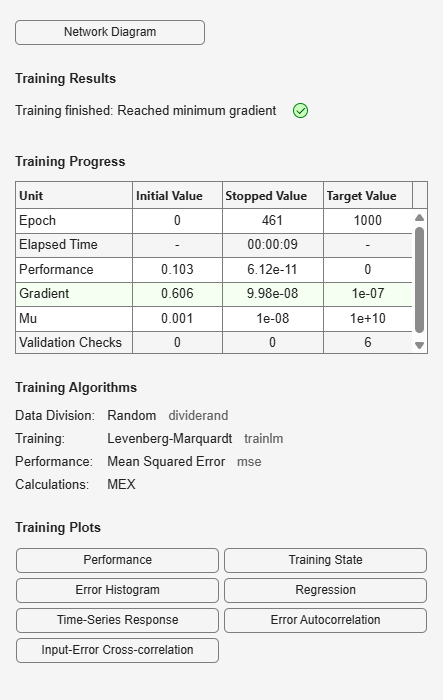# layrecnet

Layer recurrent neural network

## Syntax

``layrecnet(layerDelays,hiddenSizes,trainFcn)``

## Description

example

````layrecnet(layerDelays,hiddenSizes,trainFcn)` takes these arguments: Row vector of increasing 0 or positive delays, `layerDelays`Row vector of one or more hidden layer sizes, `hiddenSizes`Backpropagation training function, `trainFcn` and returns a layer recurrent neural network.Layer recurrent neural networks are similar to feedforward networks, except that each layer has a recurrent connection with a tap delay associated with it. This allows the network to have an infinite dynamic response to time series input data. This network is similar to the time delay (`timedelaynet`) and distributed delay (`distdelaynet`) neural networks, which have finite input responses.```

## Examples

collapse all

This example shows how to use a layer recurrent neural network to solve a simple time series problem.

```[X,T] = simpleseries_dataset; net = layrecnet(1:2,10); [Xs,Xi,Ai,Ts] = preparets(net,X,T); net = train(net,Xs,Ts,Xi,Ai); view(net) Y = net(Xs,Xi,Ai); perf = perform(net,Y,Ts) ```
```perf = 6.1239e-11 ```## Input Arguments

collapse all

Zero or positive input delays, specified as an increasing row vector.

Sizes of the hidden layers, specified as a row vector of one or more elements.

Training function name, specified as one of the following.

Training FunctionAlgorithm
`'trainlm'`

Levenberg-Marquardt

`'trainbr'`

Bayesian Regularization

`'trainbfg'`

BFGS Quasi-Newton

`'trainrp'`

Resilient Backpropagation

`'trainscg'`

`'traincgb'`

`'traincgf'`

`'traincgp'`

`'trainoss'`

One Step Secant

`'traingdx'`

`'traingdm'`

`'traingd'`

Example: For example, you can specify the variable learning rate gradient descent algorithm as the training algorithm as follows: `'traingdx'`

For more information on the training functions, see Train and Apply Multilayer Shallow Neural Networks and Choose a Multilayer Neural Network Training Function.

Data Types: `char`